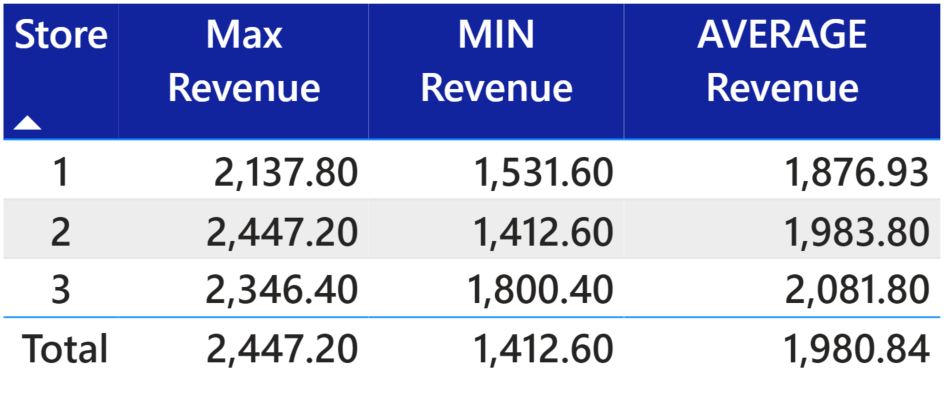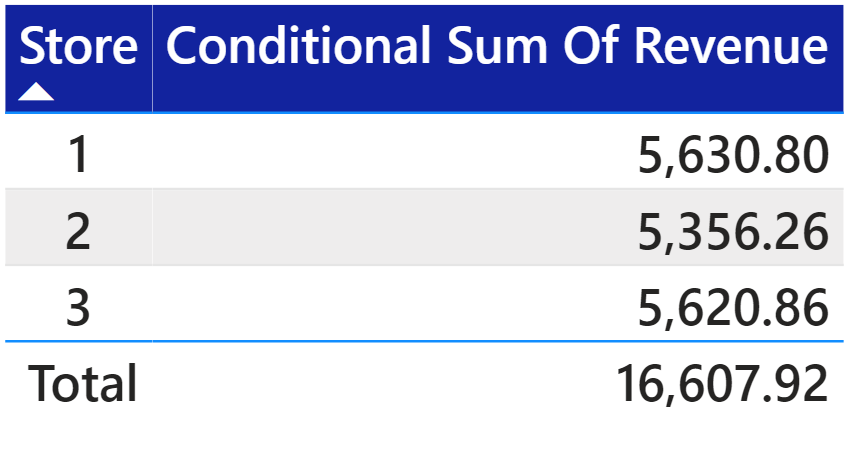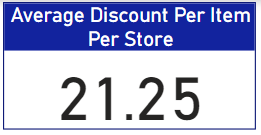Search
•Vahid

# Why my measure returns the wrong total? How to fix that?

This is a common issue that a measure returns the correct value in a table or matrix visuals, but the total value is not what you want! Every day I see some posts in the Power BI community related to the total or subtotal issues, so I decided to write this post to explain why measure returns the wrong total and how to fix it to get the expected total.

In this article, I split this issue into three categories based on shared posts on the Power BI community:

1. Aggregation or Math and Trig Functions (MAX, MIN, AVERAGE, ROUNDUP)

2. Use Condition in a Measure

3. Blank value

Sample Data:### Aggregation or Math and Trig Functions

Below measures can be used to calculate the MAX, MIN, and AVERAGE Revenue in Power BI for each store:

Max Revenue = MAX ( 'Table'[Revenue] )

MIN Revenue = MIN ( 'Table'[Revenue] ) AVERAGE Revenue = AVERAGE ( 'Table'[Revenue] )

the output after adding the store column and measures to the table visual is as follow:As you see in the image, the total values are the MAX, MIN, and AVERAGE of all individual rows in the data table, and it's not the sum of the aggregated values visible in the visual. For example, the total of the Average Revenue is the average of all Revenue values in the data table, disregarding the average value for each Store. In this case, the total is not wrong, but it's not the expected value. To update these measures to have the sum of the visible values in the visual, we need to use the IF statement with HASONEVALUE, SUMMARIZE, and SUMX.

HASONEVALUE: Returns TRUE when the context for columnName has been filtered down to one distinct value only. Otherwise is FALSE. SUMMARIZE: Returns a summary table for the requested totals over a set of groups.

SUMX: Returns the sum of an expression evaluated for each row in a table. To better understand HASONEVALUE, if I create a measure HASONEVALUE ('Table'[Store]) and add it to the table visual, it will return True for each store line in the table False for the Total line. I used the Store column in the HASONEVALUE because the table is grouped by store column.So now we can split the measures into two sides based on the result of the HASONEVALUE, when the result is true and when it's false, and it's accessible by using the IF function.

IF(<logical_test>, <value_if_true>[, <value_if_false>])

The only issue in the previous measures was wrong total values where the result of the HASONEVALUE is false, so we can use the MAX, MIN, and AVERAGE same as we used before in the <value_if_true> section of IF statement and use the SUMX and SUMMARIZE in the <value_if_false> section.

To make the SUMMARIZE output clear, first, let's review this function's parameters:

SUMMARIZE (<table>, <groupBy_columnName>[, <groupBy_columnName>]…[, <name>, <expression>]…)

Table: Any DAX expression that returns a table of data. In this case, I used the Table in this parameter (name of my sample data table in the Power BI is Table).

groupBy_ColumnName: (Optional) The qualified name of an existing column is used to create summary groups based on the values found in it. You can use more than one column to group the output based on those columns. This parameter cannot be an expression. I used Table[Store] to group the table in the store level (store column groups the output visual).

Name: The name given to the new or summarize column, enclosed in double-quotes.

Expression: Any DAX expression that returns a single scalar value, where the expression is to be evaluated multiple times (for each row/context).

For instance, if we want to summarize the sample data table at the Store level with MAX values of each Store, we can use the following DAX code:and if we use the new table and the new column in the SUMX, we will find the sum of the MAX values of each Store:Now we have all required DAX expressions (True and False) to update our measures with IF statement, so the updated measures are as follow:

Max Revenue = IF( HASONEVALUE( 'Table'[Store] ), MAX( 'Table'[Revenue] ), SUMX( SUMMARIZE( 'Table', 'Table'[Store], "MAXC", MAX( 'Table'[Revenue] ) ), [MAXC] ) )

MIN Revenue = IF ( HASONEVALUE ( 'Table'[Store] ), MIN ( 'Table'[Revenue] ), SUMX ( SUMMARIZE ( 'Table', 'Table'[Store], "MINC", MIN ( 'Table'[Revenue] ) ), [MINC] ) )

AVERAGE Revenue = IF ( HASONEVALUE ( 'Table'[Store] ), AVERAGE ( 'Table'[Revenue] ), SUMX ( SUMMARIZE ( 'Table', 'Table'[Store], "AVERAGEC", AVERAGE ( 'Table'[Revenue] ) ), [AVERAGEC] ) )

## Output:Now the total row in the table visual shows the expected values (sum of the aggregated values visible in the visual).

Also, you might face this issue while using the ROUNDUP DAX function. For instance, if we want to round up the MAX revenue of each Store and the total in the previous example, we can use the following measure:

ROUNDUP MAX Revenue = ROUNDUP([Max Revenue],0)

and the output will be as follow:Again the total value is wrong because the summation of the roundup values in the visual should be 6,933, but it's 6,932. The solution is the magical combination of HASONEVALUE, SUMMARIZE, and SUMX DAX functions. The Roundup needs to be added to the true and false section of the IF statement to find the expected total value:

ROUNDUP MAX Revenue = IF( HASONEVALUE( 'Table'[Store] ), ROUNDUP( MAX( 'Table'[Revenue] ), 0 ), SUMX( SUMMARIZE( 'Table', 'Table'[Store], "MAXC", ROUNDUP( MAX( 'Table'[Revenue] ), 0 ) ), [MAXC] ) )

Output:** Note 1 = Rather than SUMMARIZE, you can use ADDCOLUMNS

** Note 2 = Rather than HASONEVALUE you can use ISFILTERED - before that, please check this SQLBI article https://www.sqlbi.com/articles/distinguishing-hasonevalue-from-isinscope/

** Note3 = you can remove IF and HASONEVALUE from this example and use SUMMARIZE and SUMX. The reason that I used them is to review all options.

### Use Condition in a Measure

The next common issue leading to the wrong total occurs when a condition or conditions are added to the measure. For instance, if we want to apply a 10% reduction when the total revenue of each store is more than 5800, the DAX expression can be like this:

Conditional Sum Of Revenue = IF( SUM( 'Table'[Revenue] ) > 5800, SUM( 'Table'[Revenue] ) * 0.9, SUM( 'Table'[Revenue] ) )

Output:As you can see in the image, the values for the store lines are correct, but the total value is incorrect! in this case, we can use the combination of SUMMARIZE and SUMX DAX functions. Conditional Sum Of Revenue = SUMX( SUMMARIZE( 'Table', 'Table'[Store], "SUMC", IF( SUM( 'Table'[Revenue] ) > 5800, SUM( 'Table'[Revenue] ) * 0.9, SUM( 'Table'[Revenue] ) ) ), [SUMC] ) Output:### Blank Value

If your table has some blank value in some columns, then those blank items can cause some issues in the result of your measures. For instance, in the Sample Data Table, a Discount column has value for some rows, and the rest are blank. If we want to calculate the discount per item (Items with Discount) for each Store, then the first formula we might use is as below:

Discount Per Item = sum('Table'[Discount])/sum('Table'[Quantity])

Output:In this case, not only the result for stores is wrong, but also the total value is wrong. This issue is because of those blank rows; for example, in the store 1 group, only location B has a discount value that is 242 with 11 in the Quantity column, so the result should be 242/11 = 22.

Let's debug the previous measure. First, the DAX expression needs to be changed, and we need to use some variables.

Discount Per Item = Var _Discount = sum('Table'[Discount]) Var _QTY = sum('Table'[Quantity]) return _Discount/_QTY

Then we can check the result of each variable to find the issue.

Check the _Discount variable result:

Discount = Var _Discount = sum('Table'[Discount]) Var _QTY = sum('Table'[Quantity]) return _Discount

Output:it seems the result of _Discount is correct, so let's check the _QTY:

Sum of the QTY with discount = Var _Discount = sum('Table'[Discount]) Var _QTY = sum('Table'[Quantity]) return _QTY

Output:As you see in the above image, the _QTY = sum('Table'[Quantity]) will return the total quantities for each Store, but we need the total quantity for each Store with a discount.

So we can add a filter to the measure to remove those rows without a discount amount from the calculation.

Sum of the QTY with discount = VAR _Discount = SUM ( 'Table'[Discount] ) VAR _QTY = CALCULATE ( SUM ( 'Table'[Quantity] ), FILTER ( 'Table', 'Table'[Discount] > 0 ) ) RETURN _QTY

Output:Now the result for _QTY is correct, so we can calculate the Discount Per Item with the new formula for _QTY:

Discount Per Item = VAR _Discount = SUM ( 'Table'[Discount] ) VAR _QTY = CALCULATE ( SUM ( 'Table'[Quantity] ), FILTER ( 'Table', 'Table'[Discount] > 0 ) ) RETURN _Discount / _QTY

Output:The result is correct this time, even for the total! The total discount is 2492, and the total quantity with discount is 120, so 2492/120 is 20.77.

But how can we find the average discount per item per store?

We can use the AVERAGEX and SUMMARIZE along with previous measure [Discount Per Item] to find the result:

Average Discount Per Item Per Store = AVERAGEX ( SUMMARIZE ( 'Table', 'Table'[Store], "DPI", [Discount Per Item] ), [DPI] )

Output:### Sample File:

Why the total value of my DAX measure is wrong
.zip